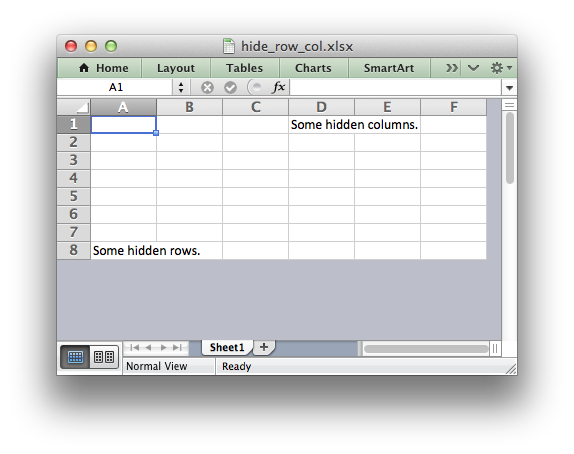# Example: Hiding Rows and Columns#

This program is an example of how to hide rows and columns in XlsxWriter.

An individual row can be hidden using the `set_row()` method:

```worksheet.set_row(0, None, None, {'hidden': True})
```

However, in order to hide a large number of rows, for example all the rows after row 8, we need to use an Excel optimization to hide rows without setting each one, (of approximately 1 million rows). To do this we use the `set_default_row()` method.

Columns don’t require this optimization and can be hidden using `set_column()`.```###############################################################################
#
# Example of how to hide rows and columns in XlsxWriter. In order to
# hide rows without setting each one, (of approximately 1 million rows),
# Excel uses an optimizations to hide all rows that don't have data.
#
# Copyright 2013-2023, John McNamara, jmcnamara@cpan.org
#
import xlsxwriter

workbook = xlsxwriter.Workbook("hide_row_col.xlsx")

# Write some data.
worksheet.write("D1", "Some hidden columns.")
worksheet.write("A8", "Some hidden rows.")

# Hide all rows without data.
worksheet.set_default_row(hide_unused_rows=True)

# Set the height of empty rows that we do want to display even if it is
# the default height.
for row in range(1, 7):
worksheet.set_row(row, 15)

# Columns can be hidden explicitly. This doesn't increase the file size..
worksheet.set_column("G:XFD", None, None, {"hidden": True})

workbook.close()
```